# STM32Cube. STM32 and ADC (Analog-to-digital converter).

Hi to all visitors of our site!

Today I’d like to talk about the ADC peripheral module and it’s configuration via STM32CubeMx.Before speaking about the aspects of configuration the project, I’d like to say some words about the challenge of this article 🙂 Let’s toggle leds on the development board (STM32F4-Discovery) depending on the input voltage at the special pin. Since the input power of the STM32 microcontrollers equals 3.3 V, we can measure any voltage between 0 and 3.3 V with the help of the ADC. So, let’s set the following intervals:

• 0 V < U < 1 V – only one led is turned on
• 1 V < U < 2 V – two leds are turned on
• 2 V < U < 3 V – three leds are turned on
• 3 V < U  – all leds are turned on (there are four leds on the development board).

So, first of all, we should create the new project in the STM32Cube framework and enable five GPIOs (four of them are connected to the leds and work in “General Output” mode, and the fifth is an ADC input). For this project I decided to use the second channel of the ADC1 (PA2 pin):We should also check is this ADC channel is enabled at the “Peripherals” tab: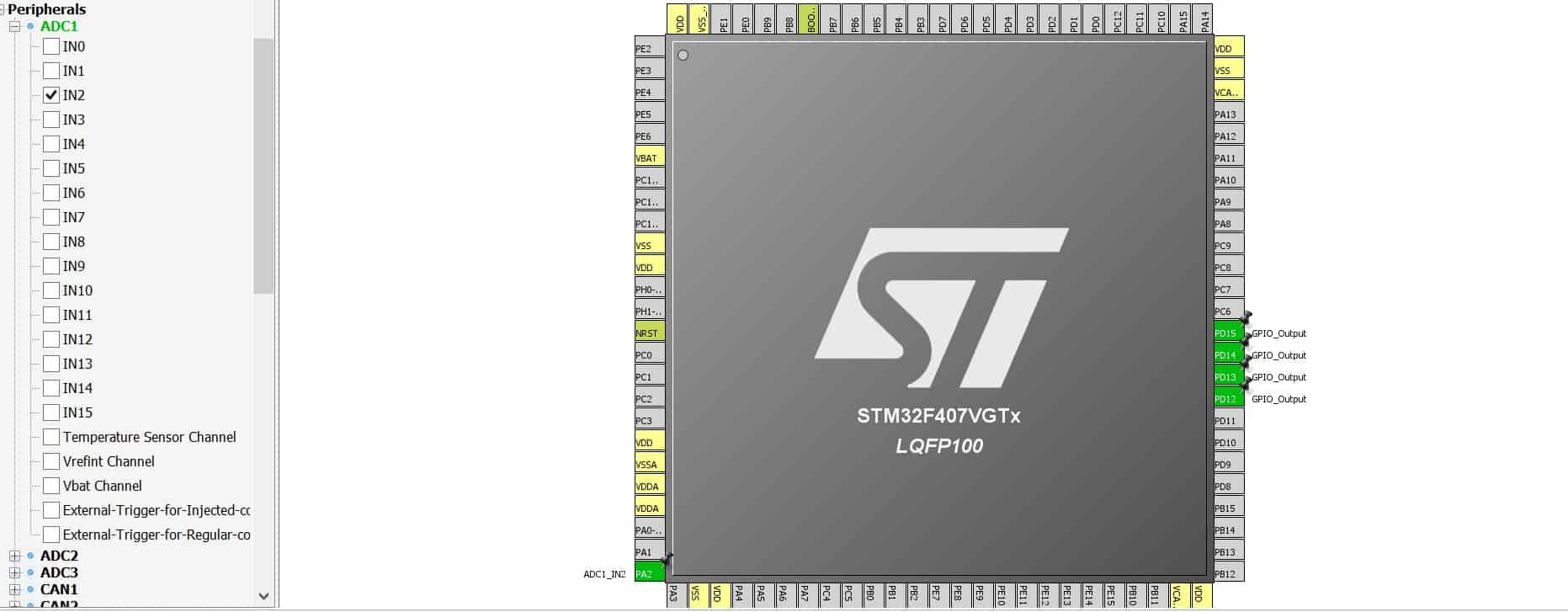Then, if we procced to the “Configuration” tab we’ll see that ADC1 extra settings have become available: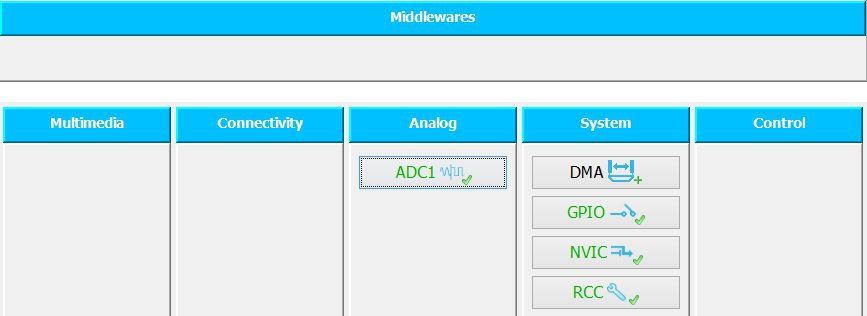If we click on the ADC1 button, a new window, where the proper mode and other features can be set, will appear: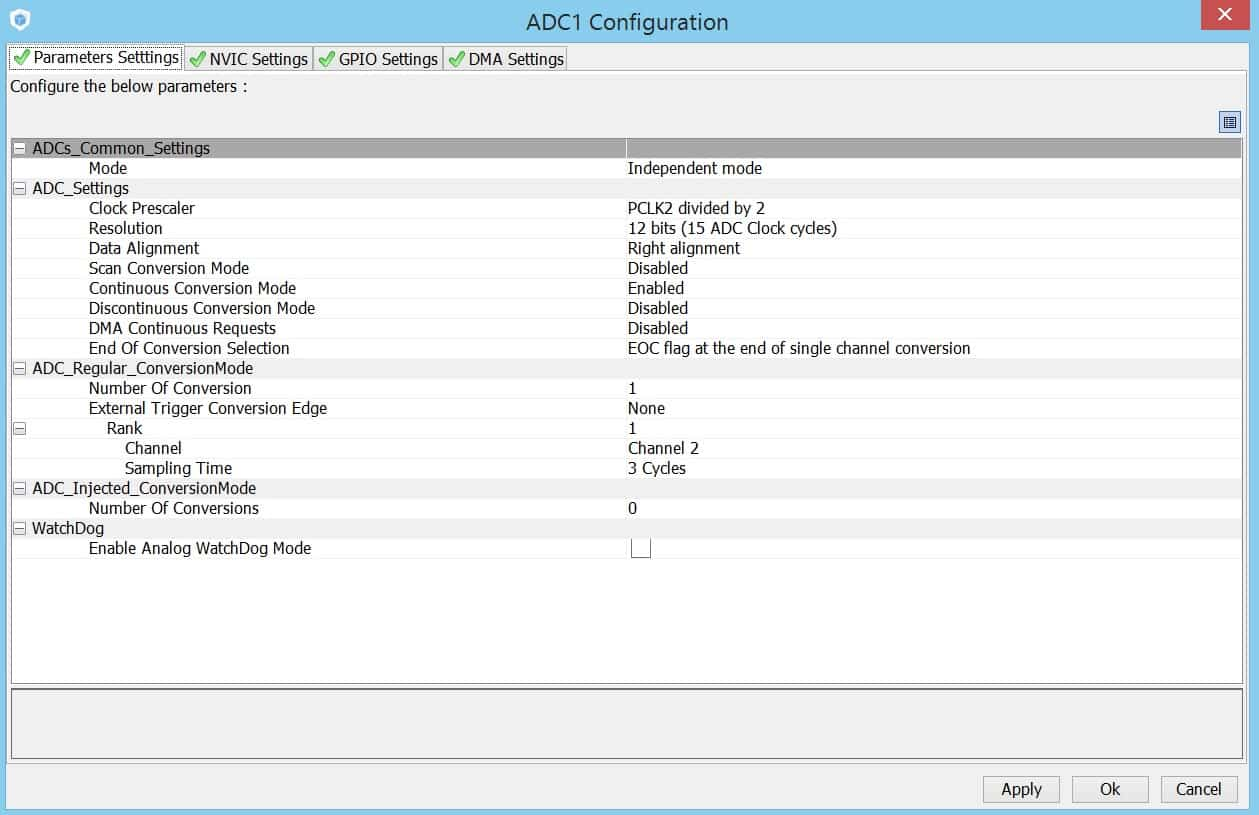After setting the parameters we can start the code generation process. And next to this we should open the project in IDE and add some code 🙂

STM32Cube takes care about everything that connected with the configuration and initialization, but other actions, such as led toggling and ADC measurement, we should realize ourselves. Firstly, declare the variable where the ADC result’ll be kept:

```/* USER CODE BEGIN PV */ uint32_t adcResult = 0;   /* USER CODE END PV */```

Secondly, we declare the voltage threshold values:

```/* USER CODE BEGIN 0 */ #define ADC_0V_VALUE 0 #define ADC_1V_VALUE 1241 #define ADC_2V_VALUE 2482 #define ADC_3V_VALUE 3723   /* USER CODE END 0 */```

The ADC peripheral result in STM32 is 12 bit. Thus, the maximum value (when the input voltage equals 3.3 V) is 4095 (0b111111111111). If the voltage at the input is 1 V, we’ll get the following result: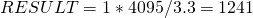If the voltage is 2 V the result’ll be: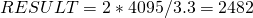Now all these definitions are clear 🙂

In function main() we should enable the ADC1 peripheral and then start the conversion. Since the conversion is finished we should analyze the result value and turn on the proper number of leds:

```int main(void) { while(1) { HAL_ADC_Start(&hadc1);   HAL_ADC_PollForConversion(&hadc1, 100);   adcResult = HAL_ADC_GetValue(&hadc1);   HAL_ADC_Stop(&hadc1);   if ((adcResult >= ADC_0V_VALUE) && (adcResult < ADC_1V_VALUE)) { HAL_GPIO_WritePin(GPIOD, GPIO_PIN_12, GPIO_PIN_SET); HAL_GPIO_WritePin(GPIOD, GPIO_PIN_13, GPIO_PIN_RESET); HAL_GPIO_WritePin(GPIOD, GPIO_PIN_14, GPIO_PIN_RESET); HAL_GPIO_WritePin(GPIOD, GPIO_PIN_15, GPIO_PIN_RESET); }   if ((adcResult >= ADC_1V_VALUE) && (adcResult < ADC_2V_VALUE)) { HAL_GPIO_WritePin(GPIOD, GPIO_PIN_12, GPIO_PIN_SET); HAL_GPIO_WritePin(GPIOD, GPIO_PIN_13, GPIO_PIN_SET); HAL_GPIO_WritePin(GPIOD, GPIO_PIN_14, GPIO_PIN_RESET); HAL_GPIO_WritePin(GPIOD, GPIO_PIN_15, GPIO_PIN_RESET); }   if ((adcResult >= ADC_2V_VALUE) && (adcResult < ADC_3V_VALUE)) { HAL_GPIO_WritePin(GPIOD, GPIO_PIN_12, GPIO_PIN_SET); HAL_GPIO_WritePin(GPIOD, GPIO_PIN_13, GPIO_PIN_SET); HAL_GPIO_WritePin(GPIOD, GPIO_PIN_14, GPIO_PIN_SET); HAL_GPIO_WritePin(GPIOD, GPIO_PIN_15, GPIO_PIN_RESET); }   if (adcResult >= ADC_3V_VALUE) { HAL_GPIO_WritePin(GPIOD, GPIO_PIN_12, GPIO_PIN_SET); HAL_GPIO_WritePin(GPIOD, GPIO_PIN_13, GPIO_PIN_SET); HAL_GPIO_WritePin(GPIOD, GPIO_PIN_14, GPIO_PIN_SET); HAL_GPIO_WritePin(GPIOD, GPIO_PIN_15, GPIO_PIN_SET); } }     }```

After compiling the project and programming the MCU we can see that one/two/three or four leds are turned on depending on the input voltage.

To conclude this post I can say that we’ll continue studying the STM32Cube in the future articles. Subscribe to MicroTechnics.ru and visit our site again! We’ll be glad to see you! 🙂

### Similar posts:

#### Like this post? Suggest to friends!

4 thoughts on “STM32Cube. STM32 and ADC (Analog-to-digital converter).
1.Sheetal on said:

•Aveal on said:
2.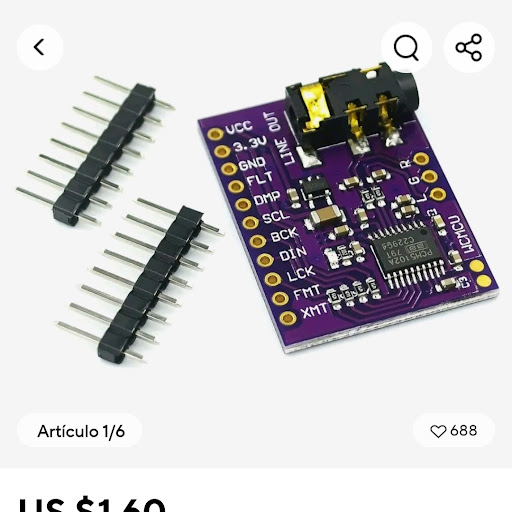Matias Ceron on said:
•Aveal on said: